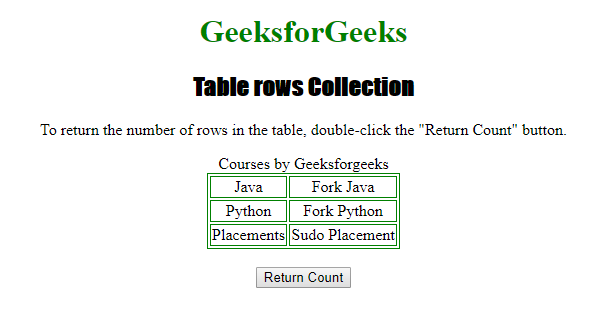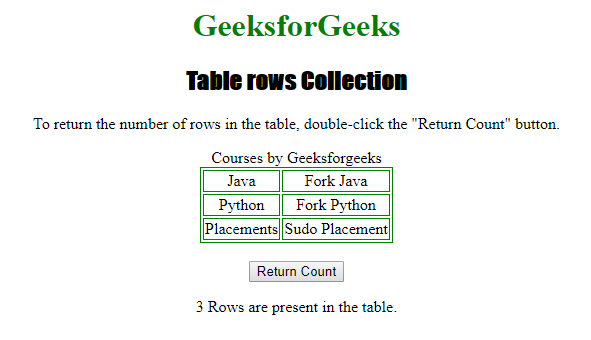# HTML | DOM Table rows Collection

The Table rows collection is used for returning the collection of all the <tr> elements in a table. The sequence of the <tr> elements are sorted in the same way as their position in the source code.

Syntax

`tableObject.rows`

Properties

• length : It is used to return the number of <tr> elements in the collection.

Methods

• [index] : It is used for returning the <tr> element from the collection with a specified index.
• item(index) : It is also used for returning the <tr> element from the collection with a specified index.
• namedItem(id) : It is also used for returning the <tr> element from the collection with a specified id.

Below program illustrates the Table rows collection :
Example: Finding out the number of rows in a table.

 ` ` `<``html``> ` ` `  `<``head``> ` `    ``<``title``>Table rows Collection in HTML ` `  `` ` `    ``<``style``> ` `        ``table, ` `        ``td { ` `            ``border: 1px solid green; ` `        ``} ` `         `  `        ``h1 { ` `            ``color: green; ` `        ``} ` `         `  `        ``h2 { ` `            ``font-family: Impact; ` `        ``} ` `         `  `        ``body { ` `            ``text-align: center; ` `        ``} ` `    `` ` ` ` ` `  `<``body``> ` ` `  `    ``<``h1``>GeeksforGeeks ` `    ``<``h2``>Table rows Collection ` ` `  `    ``<``p``>To return the number of rows in the table, ` `      ``double-click the "Return Count" button. ` ` `  `    ``<``table` `id``=``"Courses"` `align``=``"center"``> ` `        ``<``caption``>Courses by Geeksforgeeks ` `        ``<``tr``> ` `            ``<``td``>Java ` `            ``<``td``>Fork Java ` `        `` ` `        ``<``tr``> ` `            ``<``td``>Python ` `            ``<``td``>Fork Python ` `        `` ` `        ``<``tr``> ` `            ``<``td``>Placements ` `            ``<``td``>Sudo Placement ` `        `` ` `    `` ` `    ``<``br``> ` ` `  `    ``<``button` `ondblclick``=``"tr()"``>Return Count ` ` `  `    ``<``p` `id``=``"test"``> ` ` `  `    ``<``script``> ` `        ``function tr() { ` `           `  `            ``// number of rows. ` `            ``var c = document.getElementById( ` `              ``"Courses").rows.length; ` `            ``document.getElementById("test").innerHTML =  ` `              ``c + " Rows are present in the table."; ` `        ``} ` `    `` ` ` `  ` ` ` `  ` `

Output:

Before clicking the button:After clicking the button:Supported Browsers:

• Apple Safari
• Internet Explorer
• Firefox